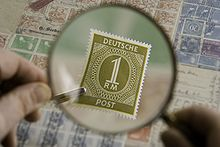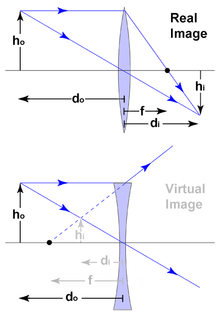# Magnification

﻿
MagnificationThe stamp appears larger with the use of a magnifying glass

Magnification is the process of enlarging something only in appearance, not in physical size. This enlargement is quantified by a calculated number also called "magnification". When this number is less than one it refers to a reduction in size, sometimes called "minification" or "de-magnification".

Typically magnification is related to scaling up visuals or images to be able to see more detail, increasing resolution, using optics, printing techniques, or digital processing. In all cases, the magnification of the image does not change the perspective of the image.

## Examples of magnification

• A magnifying glass which uses a positive (convex) lens to make things look bigger by allowing the user to hold them closer to his eye.
• A telescope which uses its large objective lens to create an image of a distant object and then allows the user to examine the image closely with a smaller eyepiece lens thus making the object look larger.
• A microscope which makes a small object appear as a much larger object at a comfortable distance for viewing. A microscope is similar in layout to a telescope except that the object being viewed is close to the objective, which is usually much smaller than the eyepiece.
• a slide projector which projects a large image of a small slide on a screen.

## Magnification as a number (optical magnification)

Optical magnification is the ratio between the apparent size of an object (or its size in an image) and its true size, and thus it is a dimensionless number.

• Linear or transverse magnification — For real images, such as images projected on a screen, size means a linear dimension (measured, for example, in millimeters or inches).
• Angular magnification — For optical instruments with an eyepiece, the linear dimension of the image seen in the eyepiece (virtual image in infinite distance) cannot be given, thus size means the angle subtended by the object at the focal point (angular size). Strictly speaking, one should take the tangent of that angle (in practice, this makes a difference only if the angle is larger than a few degrees). Thus, angular magnification is given by:$\mathrm{MA}=\frac{\tan \varepsilon}{\tan \varepsilon_0}$,
where ε0 is the angle subtended by the object at the front focal point of the objective and ε is the angle subtended by the image at the rear focal point of the eyepiece.
• Example: The angular size of the full moon is 0.5°, in binoculars with 10x magnification it appears to subtend an angle of 5°, which is roughly 1/10 of the field of view of typical eyepieces.
By convention, for magnifying glasses and optical microscopes, where the size of the object is a linear dimension and the apparent size is an angle, the magnification is the ratio between the apparent (angular) size as seen in the eyepiece and the angular size of the object when placed at the conventional closest distance of distinct vision: 25 cm from the eye.

Optical magnification is sometimes referred to as "power" (for example "10× power"), although this can lead to confusion with optical power.

### Calculating the magnification of optical systemsA Thin lens where black dimensions are real, grey are virtual. The direction of the arrows can be used to describe cartesian +/- signage : from the centre of the lens, left or down = negative, right or up = positive.$M = {f \over f-d_o}$
where f is the focal length and do is the distance from the lens to the object. Note that for real images, M is negative and the image is inverted. For virtual images, M is positive and the image is upright.
With di being the distance from the lens to the image, hi the height of the image and ho the height of the object, the magnification can also be written as:$M = -{d_i \over d_o} = {h_i \over h_o}$
Note again that a negative magnification implies an inverted image.
• Photography: The image recorded by a photographic film or image sensor is always a real image and is usually inverted. When measuring the height of an inverted image using the cartesian sign convention (where the x-axis is the optical axis) the value for hi will be negative, and as a result M will also be negative. However, the traditional sign convention used in photography is "real is positive, virtual is negative". Therefore in photography: Object height and distance are always real and positive. When the focal length is positive the image's height, distance and magnification are real and positive. Only if the focal length is negative, the image's height, distance and magnification are virtual and negative. Therefore the photographic magnification formulae are traditionally presented as:$M = {d_i \over d_o} = {h_i \over h_o} = {f \over d_o-f} = {d_i-f \over f}$
• Telescope: The angular magnification is given by$M= {f_o \over f_e}$
where fo is the focal length of the objective lens and fe is the focal length of the eyepiece.
• Magnifying glass: The maximum angular magnification (compared to the naked eye) of a magnifying glass depends on how the glass and the object are held, relative to the eye. If the lens is held at a distance from the object that its front focal point is on the object being viewed, the relaxed eye (focused to infinity) can view the image with angular magnification$\mathrm{MA}={25\ \mathrm{cm}\over f}\quad$
Here, f is the focal length of the lens in centimeters. The constant 25 cm is an estimate of the "near point" distance of the eye—the closest distance at which the healthy naked eye can focus. In this case the angular maginification is independant from the distance kept between the eye and the magnifying glass.
If instead the lens is held very close to the eye and the object is placed closer to the lens than its focal point so that the observer focuses on the near point, a larger angular magnification can be obtained, approaching$\mathrm{MA}={25\ \mathrm{cm}\over f}+1\quad$
A different interpretation of the working of the latter case is that the magnifying glass changes the diopter of the eye (making it myopic) so that the object can be placed closer to the eye resulting in a larger angular magnification.
• Microscope: The angular magnification is given by$\mathrm{MA}=M_o \times M_e$
where Mo is the magnification of the objective and Me the magnification of the eyepiece. The magnification of the objective depends on its focal length fo and on the distance d between objective back focal plane and the focal plane of the eyepiece (called the tube length):$M_o={d \over f_o}$.
The magnification of the eyepiece depends upon its focal length fe and calculated by the same equation as that of a magnifying glass (above).

Note that both astronomical telescopes as well as simple microscopes produce an inverted image, thus the equation for the magnification of a telescope or microscope is often given with a minus sign[citation needed].

### Measurement of telescope magnification

Measuring the actual angular magnification of a telescope is difficult, but it is possible to use the reciprocal relationship between the linear magnification and the angular magnification, since the linear magnification is constant for all objects.

The telescope is focused correctly for viewing objects at the distance for which the angular magnification is to be determined and then the object glass is used as an object the image of which is known as the Ramsden disc. The diameter of this may be measured using an instrument known as a Ramsden dynameter which consists of a Ramsden eyepiece with micrometer hairs in the back focal plane. This is mounted in front of the telescope eyepiece and used to evaluate the diameter of the Ramsden disc. This will be much smaller than the object glass diameter, which gives the linear magnification (actually a reduction), the angular magnification can be determined from

MA = 1 / M = DObjective / DRamsden

### Maximum usable magnification

With any telescope or microscope, there is a maximum magnification beyond which the image looks bigger but shows no more detail. It occurs when the finest detail the instrument can resolve is magnified to match the finest detail the eye can see. Magnification beyond this maximum is sometimes called "empty magnification".

For a good quality telescope operating in good atmospheric conditions, the maximum usable magnification is limited by diffraction. In practice it is widely considered to be 2× the aperture in millimetres or 50× the aperture in inches, so a 60mm diameter telescope has a maximum usable magnification of 120×.

With an optical microscope having a high numerical aperture and using oil immersion, the best possible resolution is 200 nm corresponding to a magnification of around 1200×. Without oil immersion, the maximum usable magnification is around 800×. For details, see limitations of optical microscopes.

Small, cheap telescopes and microscopes are sometimes supplied with eyepieces that give magnification far higher than is usable.

Wikimedia Foundation. 2010.

### Look at other dictionaries:

• Magnification — Studioalbum von Yes Veröffentlichung 2001 Label Eagle Records (U.K.) Beyond Music ( …   Deutsch Wikipedia

• Magnification — Album par Yes Sortie 11 septembre 2001 (Royaume Uni) 4 décembre 2001 (États Unis) Durée 1:00:32 Genre Rock progressif rock symphonique …   Wikipédia en Français

• Magnification — Mag ni*fi*ca tion, n. The act of magnifying; enlargement; exaggeration. [R.] [1913 Webster] …   The Collaborative International Dictionary of English

• magnification — index aggravation (exacerbation), augmentation, bombast, boom (increase), distortion, exaggeration …   Law dictionary

• magnification — (n.) early 15c., from L.L. magnificationem (nom. magnificatio), noun of action from pp. stem of magnificare (see MAGNIFY (Cf. magnify)) …   Etymology dictionary

• magnification — [mag΄nə fi kā′shən] n. [LL magnificatio] 1. a magnifying or being magnified 2. the power of magnifying 3. a magnified image, model, or representation …   English World dictionary

• magnification — /mag neuh fi kay sheuhn/, n. 1. the act of magnifying or the state of being magnified. 2. the power to magnify. Cf. power (def. 20a). 3. a magnified image, drawing, copy, etc. [1615 25; < LL magnification (s. of magnificatio). See MAGNIFY,… …   Universalium

• magnification — mag|ni|fi|ca|tion [ˌmægnıfıˈkeıʃən] n 1.) [U] the process of making something look bigger than it is at high/low etc magnification ▪ When viewed at high magnification it is clear that the crystals are quite different. ▪ greater levels of… …   Dictionary of contemporary English

• magnification — [[t]mæ̱gnɪfɪke͟ɪʃ(ə)n[/t]] magnifications 1) N UNCOUNT Magnification is the act or process of magnifying something. The man was tall, his figure shortened by the magnification of Lenny s binoculars... I find England strange and unique and… …   English dictionary

• magnification —    The term magnification is indebted to the Latin adjective magnus, which means large. It is used to denote the process by which visually perceived objects or stimuli appear to increase in size. Magnification can occur in individuals wearing… …   Dictionary of Hallucinations